# 1. Suppose a firm wants to minimize the costs associated with producing according to function: q...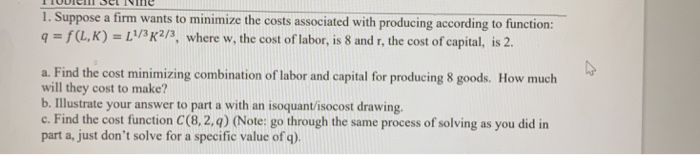1. Suppose a firm wants to minimize the costs associated with producing according to function: q = f(L,K) = [1/3,2/3, where w, the cost of labor, is 8 and r, the cost of capital, is 2. w a. Find the cost minimizing combination of labor and capital for producing 8 goods. How much will they cost to make? b. Illustrate your answer to part a with an isoquant/isocost drawing. c. Find the cost function C(8,2,q) (Note: go through the same process of solving as you did in part a, just don't solve for a specific value of q).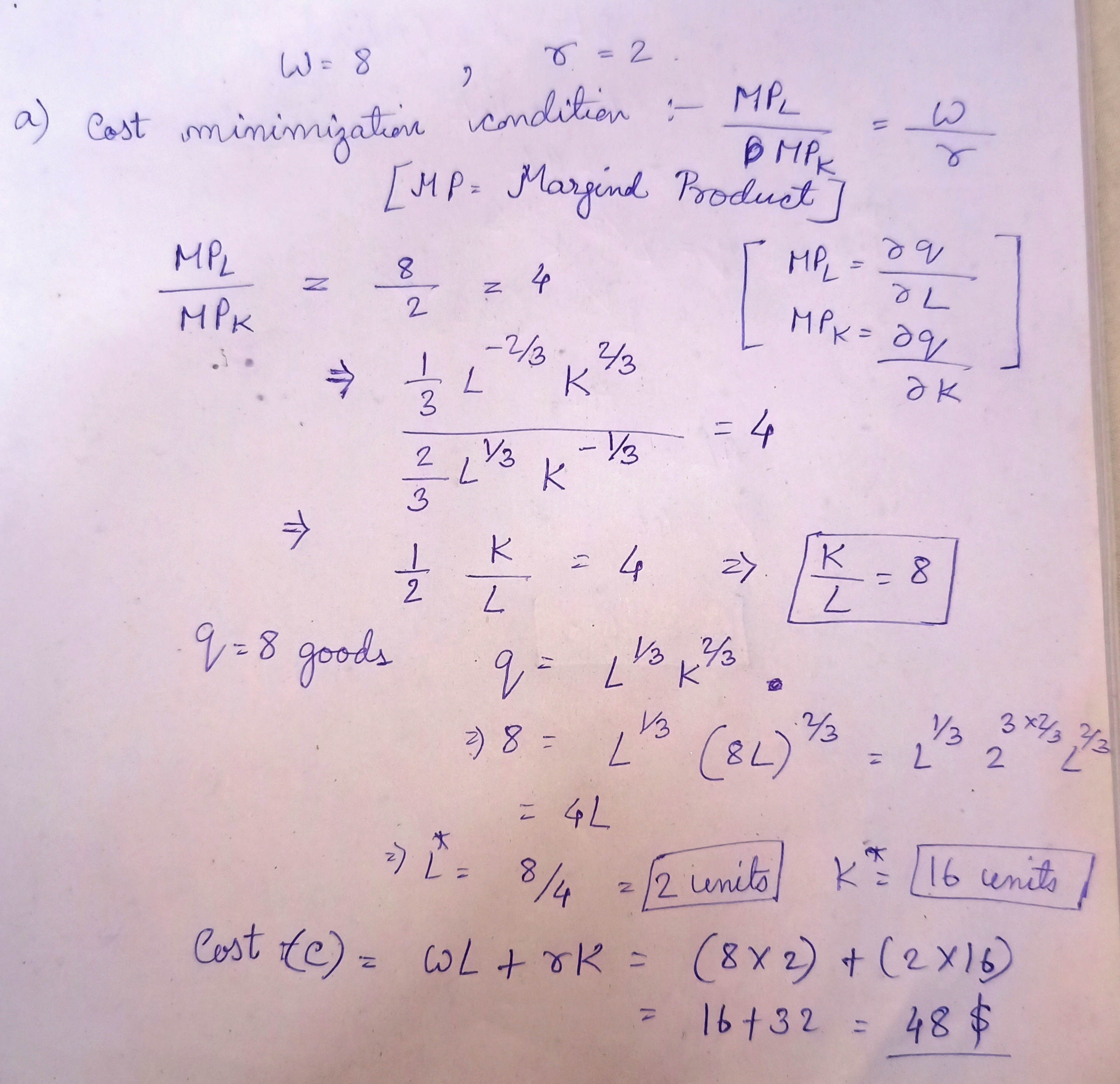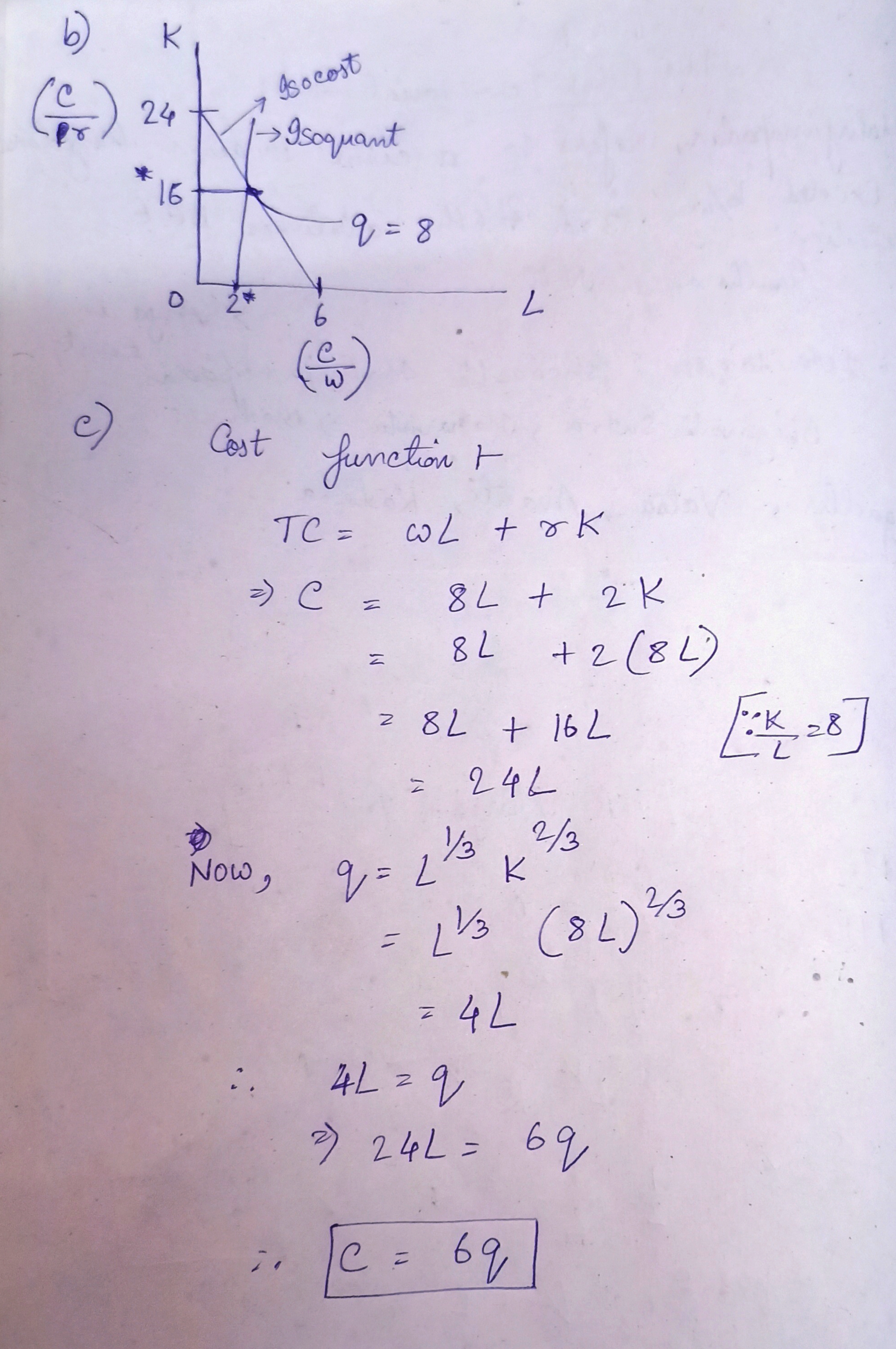#### Earn Coin

Coins can be redeemed for fabulous gifts.

Similar Homework Help Questions
• ### 2. Assume a firm can produce according to the production function: ? = ?(?, ?) =...

2. Assume a firm can produce according to the production function: ? = ?(?, ?) = ? + 3? Assume the wage, the cost of labor, is 6, and rent the cost of capital, is 12. What is the best combination of labor and capital to produce 12 units with? Illustrate your answer to part a with an isoquant/isocost drawing. If the wage decreased to \$2, would this change how the firm will produce? If so, how? 3. Assume a...

• ### 1. Suppose a firm is producing output according to Q=1001KL. A. Draw a sketch of this...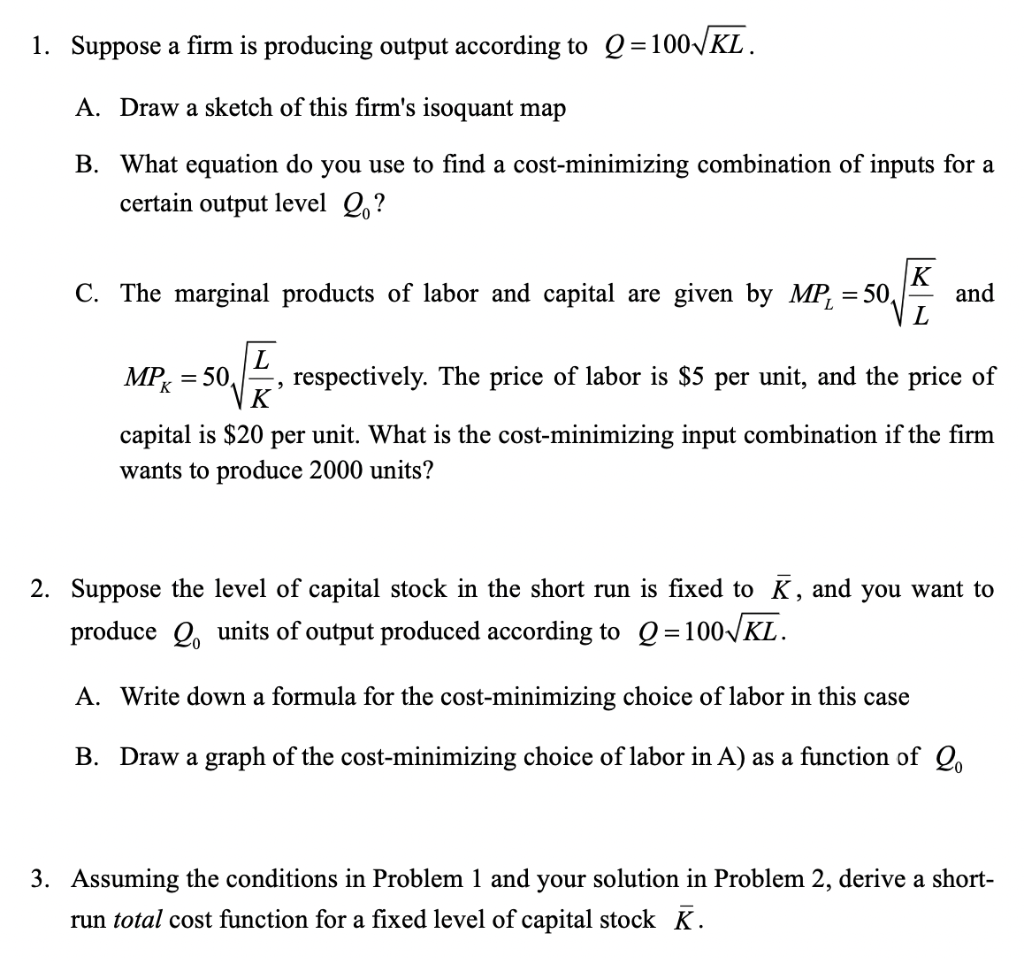1. Suppose a firm is producing output according to Q=1001KL. A. Draw a sketch of this firm's isoquant map B. What equation do you use to find a cost-minimizing combination of inputs for a certain output level Q.? K C. The marginal products of labor and capital are given by MP, = 50, and L MPK = 50, L respectively. The price of labor is \$5 per unit, and the price of K capital is \$20 per unit. What is...

• ### 3. Suppose the production of Crocs is characterized by the production function Q = LK, where...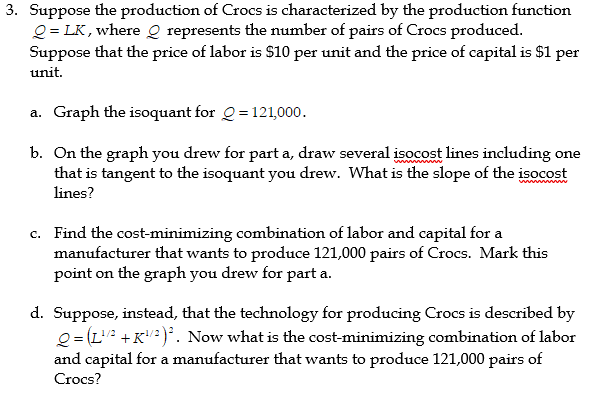3. Suppose the production of Crocs is characterized by the production function Q = LK, where represents the number of pairs of Crocs produced. Suppose that the price of labor is \$10 per unit and the price of capital is \$1 per unit. a. Graph the isoquant for Q=121,000. b. On the graph you drew for part a, draw several isocost lines including one that is tangent to the isoquant you drew. What is the slope of the isocost lines?...

• ### Suppose a firm produces an output level according to the simple production function: Q = 5...

Suppose a firm produces an output level according to the simple production function: Q = 5 L K, which implies M P L = 5 K and M P K = 5 L. Further suppose a firm must pay labor (L) a wage rate (w) of \$5 per unit, and the rental rate (r) on capital (K) is \$25 per unit. A. Find the marginal rate of technical substitution. B. Write the equation for the isocost line. What is the...

• ### Suppose a firm can use either Capital (K) or Labor (L) in a production process. The...

Suppose a firm can use either Capital (K) or Labor (L) in a production process. The firms Production function is given by Q = 5L + 15K. The price of Capital is \$20 per unit and the price of Labor is \$8 per unit. a) (4 points) What is the firm’s Total Cost function? TC(Q) = ____________________________ b) (8 points) Suppose the firm is producing 30 units of output (Q = 30). Using a graph, draw the firm’s isoquant for...

• ### 2. (12 total points) Suppose a firm can use either Capital (K) or Labor (L) in...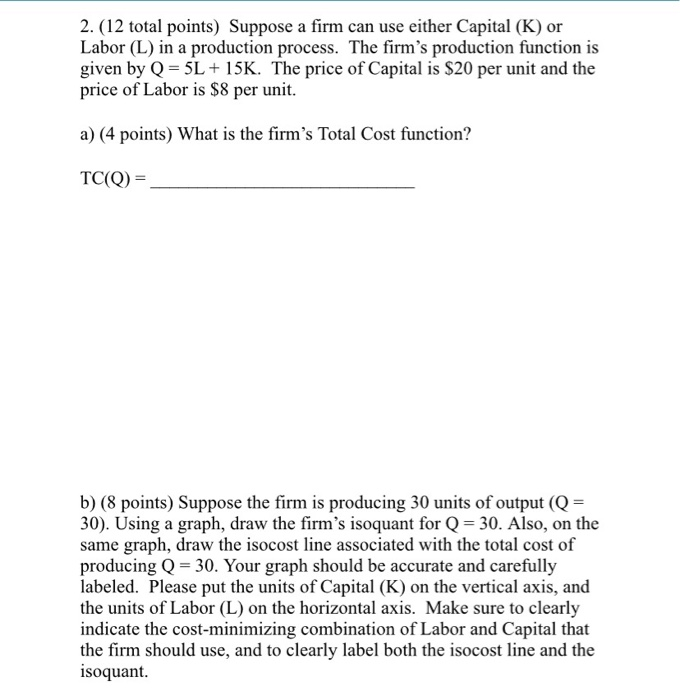2. (12 total points) Suppose a firm can use either Capital (K) or Labor (L) in a production process. The firm's production function is given by Q = 5L + 15K. The price of Capital is \$20 per unit and the price of Labor is \$8 per unit. a) (4 points) What is the firm's Total Cost function? TC(Q)= b) (8 points) Suppose the firm is producing 30 units of output (Q = 30). Using a graph, draw the firm's...

• ### a firm can minimize cost by using the combination of inputs A firm can minimize cost...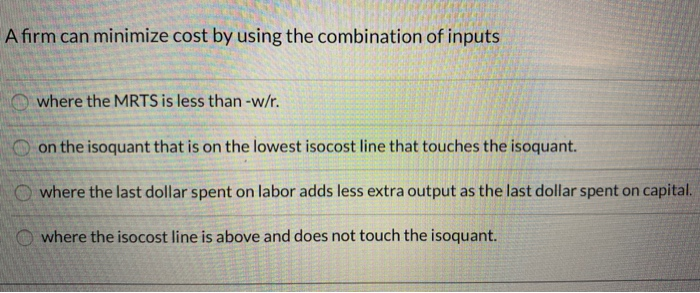a firm can minimize cost by using the combination of inputs A firm can minimize cost by using the combination of inputs where the MRTS is less than-w/r. on the isoquant that is on the lowest isocost line that touches the isoquant. where the last dollar spent on labor adds less extra output as the last dollar spent on capital. where the isocost line is above and does not touch the isoquant.

• ### 1. Suppose the production of digital cameras is characterized by the production function q F(K, L)- KL (MPL = K, MPK = L), where q represents the number of digital cameras produced. Suppose that...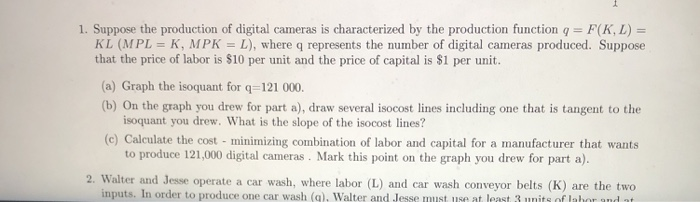1. Suppose the production of digital cameras is characterized by the production function q F(K, L)- KL (MPL = K, MPK = L), where q represents the number of digital cameras produced. Suppose that the price of labor is \$10 per unit and the price of capital is S1 per unit. (a) Graph the isoquant for q-121 000. (b) On the graph you drew for part a), draw several isocost lines including one that is tangent to the isoquant you...

• ### 2. Assume that a firm needs to produce 500 units of output and it has ability...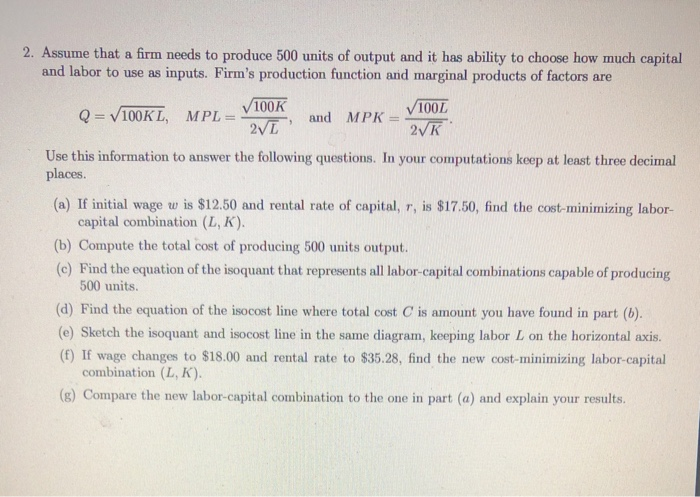2. Assume that a firm needs to produce 500 units of output and it has ability to choose how much capital and labor to use as inputs. Firm's production function and marginal products of factors are 2VL, and MPK 100L ых 100K . Use this information to answer the following questions. In your computations keep at least three decimal places. (a) If initial wage w is \$12.50 and rental rate of capital, r, is \$17.50, find the cost-minimizing labor- capital...

• ### K Isoquant: Q 70 Tangency 8 Isocost: TC - \$? 10 Figure 1. Isoquant and Isocost...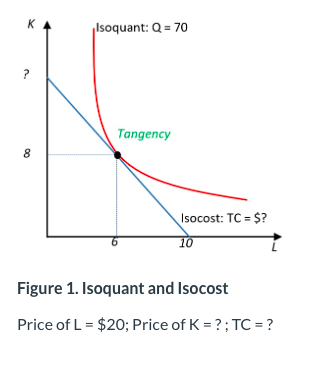K Isoquant: Q 70 Tangency 8 Isocost: TC - \$? 10 Figure 1. Isoquant and Isocost Price of L = \$20; Price of K ? ; TC ? 16. Refer to Figure 1. If the price of capital increases while the price of labor is unchanged, which of the following is most accurate? In the new cost-minimizing input combination for producing Q-70, the labor input must be lower than 6. The isoquant of Q = 70 shifts inward. It is...